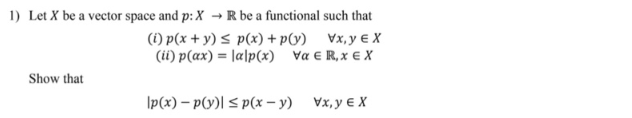## Show that IP(x) - P(y)= p(x - y) Vx, y + XTranscript:
1) Let X be a vector space and p:X → R be a functional such that (1) p(x + y) s p(x) + p(y) Vxy e X (ii) p(ax) = |a|p(x) Va € R,x € X Show that IP(x) - P(y)= p(x - y) Vx, y + X

Solved